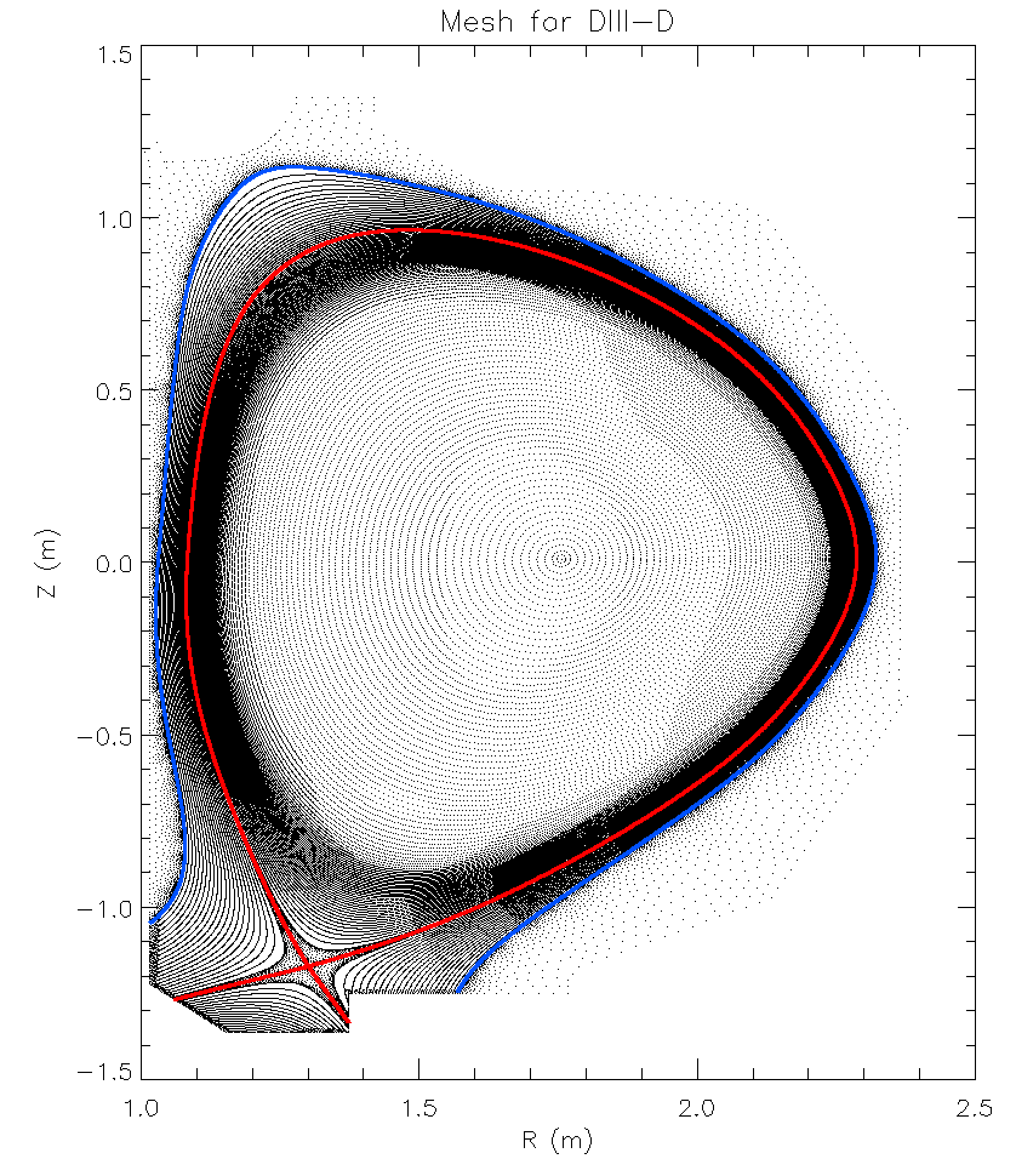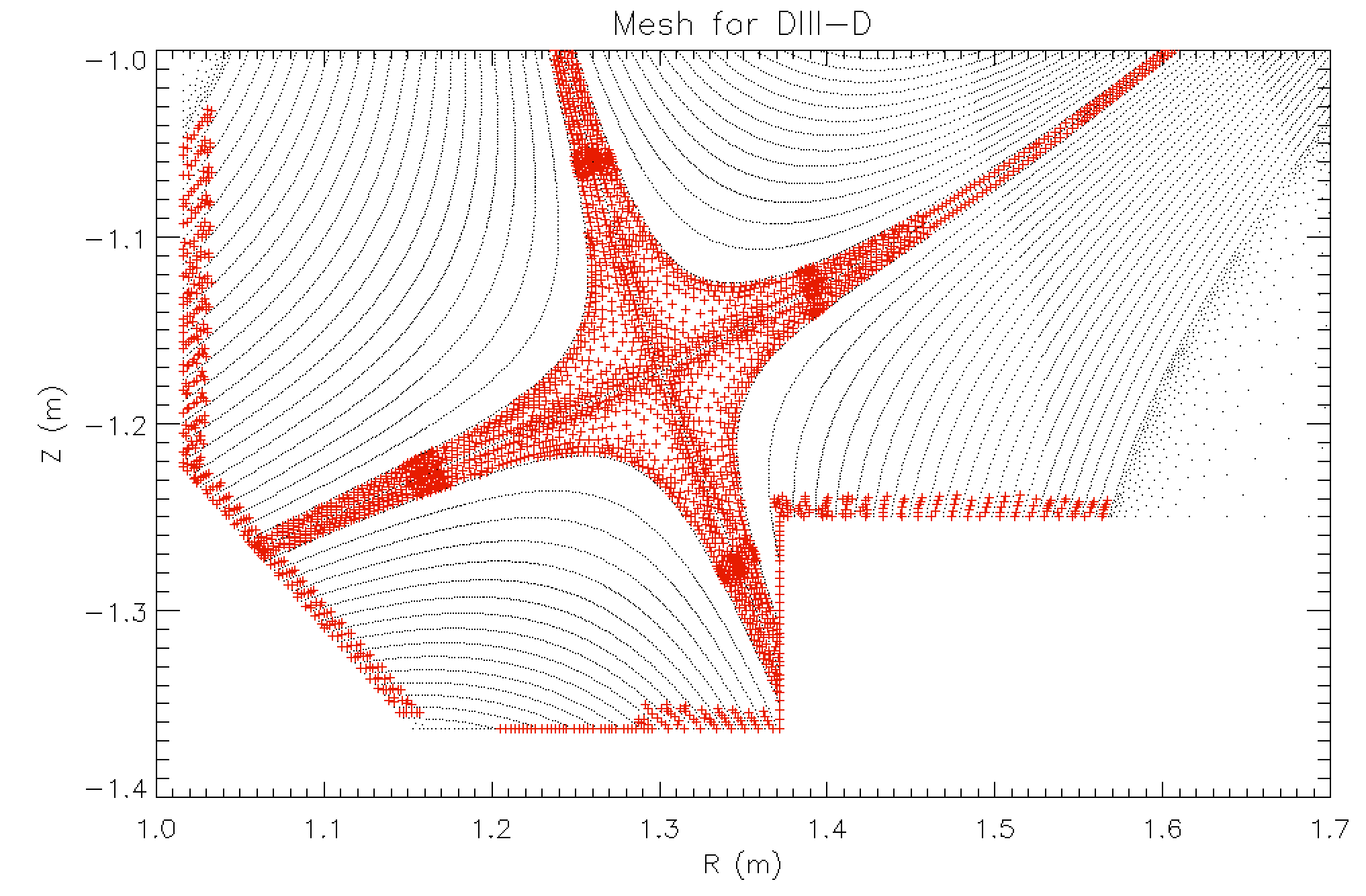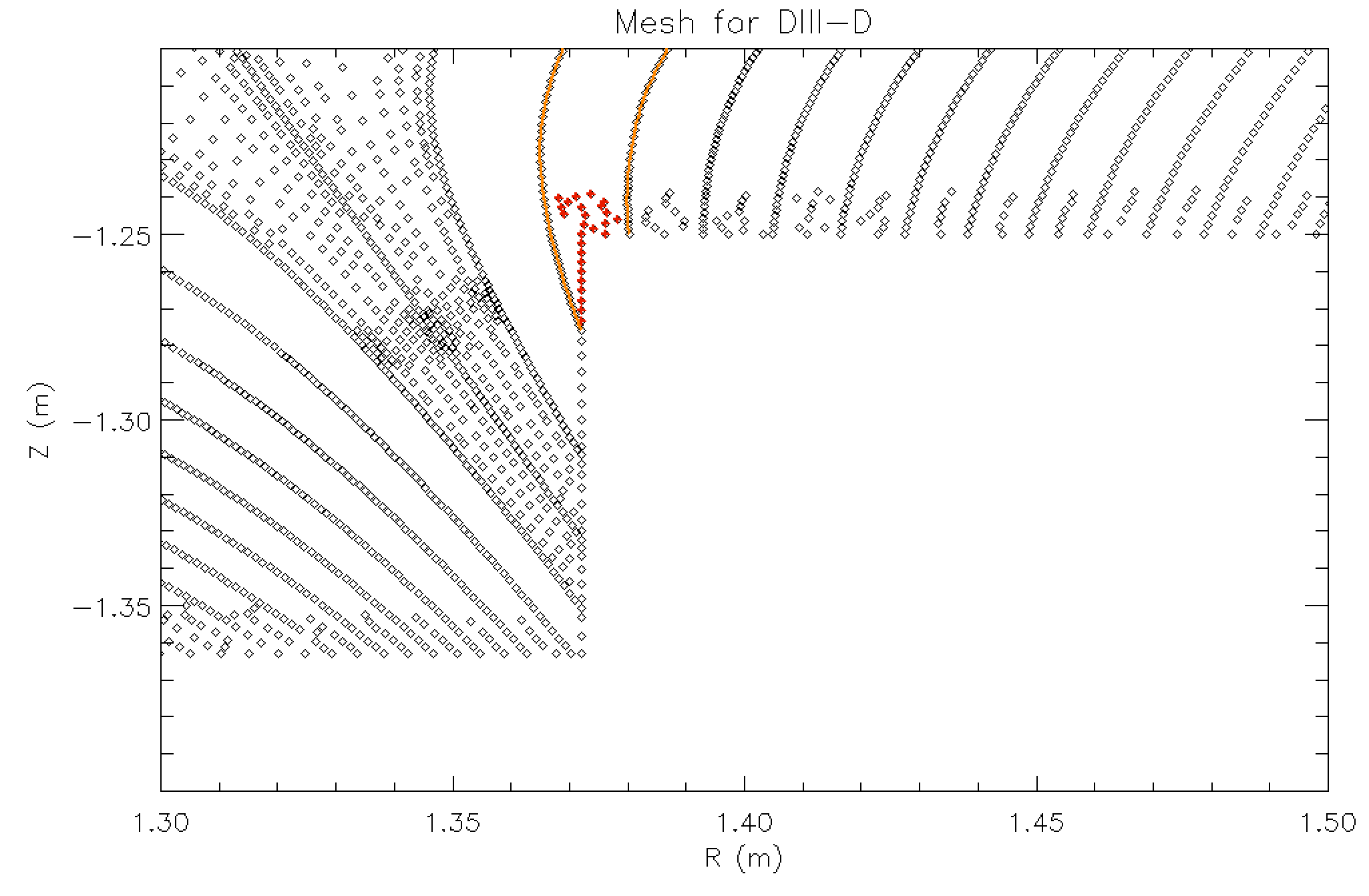# File Format of XGC Mesh Files¶

XGC requires three input files to set up its triangular solver mesh:

1. A file containing the cylindrical coordinates R and Z of the mesh vertices (the “node” file).

2. A file containing the triangle connectivity of the vertices (the “ele” file).

3. A file containing the flux-surface connectivity of the vertices (the “flx” file).

The following paragraphs describes the file format of those files.

## The “node” File¶

The “node” file starts with a header line that contains the number of vertices of the mesh, dimension (must be 2), number of attributes and number of boundary markers (0 or 1). The information is in the following format:

<number of vertices> <dimension> <number of attributes> <number of boundary markers>


XGC only supports:

• dimension = 2

• number of attributes = 0

• number of boundary markers = 1

The header line in the “node” file should look like this

<number of vertices>  2  0  1


Each of the following lines contains the R and Z coordinates of the mesh vertices, e.g.:

181162  2  0  1
1 1.218645 -1.363000 0
2 1.216197 -1.363000 0
3 1.220985 -1.363000 0
4 1.218374 -1.360182 0
5 1.220244 -1.360709 0
[...]


The vertex data is arranged in four columns. The first column is the vertex index. The second and third column are the R and Z coordinates, and the last column indicates whether the vertex is on the wall curve (1) or not (0).Fig. 1: Example of an XGC mesh. There are four distinct regions, i) the closed flux-surface region (core), ii) the scrape-off layer (SOL), iii) the private flux region, and iv) the unstructured mesh region (outside the blue surface). The separatrix, which includes the X-point and separates core, SOL and private region, is shown in red. There are cases with two X-points and two separatrices within the wall curve. In those cases, there is also a second private region.

## The “ele” file¶

The “ele” file contains the triangle connectivity of the mesh. The header line in the file contains the number of triangles, nodes per triangle and number of attributes. The information is in the following format:

<number of triangles> <nodes per triangle> <number of attributes>


XGC only supports:

• nodes per triangle = 3

• number of attributes = 0

The header line in the “ele” file should look like this

<number of triangles>  3  0


Each of the following lines lists the triangle index and the indices of the three vertices of the triangle, e.g.:

361725        3        0
1      2      1      4
2      5      1      3
3      1      5      4
4      2      7      6
5      2      8      7
6      8      2      4
7     10      3      9
[...]


## The “flx” file¶

Most vertices in XGC meshes are not really unstructured, but are aligned on a set of surfaces of constant poloidal magnetic flux (flux-surfaces or flux-curves). The minority of vertices is really unstructured. In this sense, XGC meshes are divided into two regions, the field-aligned and unstructured regions. The two regions are separated by the blue flux-surface in Fig. 1. Around the X-point and where flux-surfaces intersect with the wall curve, additional vertices that are not field-aligned have to be added to generate high-quality meshes. Those vertices are called “non-aligned vertices” in the remainder of this section. Fig. 2 shoes an example of those non-aligned vertices.Fig. 2: Nonaligned vertices are shown in red, field-aligned vertices and unstructured vertices are shown in black. The non-aligned vertices are all in the region that is otherwise filled with field-aligned mesh (inside the blue surface in Fig. 1).

Simply speaking, flux-surface averages <A> in XGC are computed by calculating the mean of A over the vertices on the same flux-surface. In many situations, it is necessary to know the value of <A> on each vertex of the mesh. This value is readily obtained for all field-aligned vertices. The value of <A> on the non-aligned vertices, on the other hand, is obtained through interpolation from the field-aligned vertices. In XGC, linear interpolation is used, which requires knowledge of the two flux-surfaces between which each non-aligned vertex lies. Fig. 3 shows an example. Two adjacent flux surfaces are shown in orange, and the non-aligned vertices between them are shown in red.The “flx” file contains lists of vertices that are on the same flux-surface, the two flux-surfaces associated with each of the non-aligned vertices, and some other information about the mesh structure.

1
1920   -1
93 23 16  0
94 -1
1   11   21   29
69137
70218  70221  70222  70223 [...]
72375  72376  72377  72378 [...]
75593  75597  75598  75599 [...]
[...]
-1
3893
11131
106 107
10822
106 107
10825
106 107
10532
-1


The first line is the number of separatrices/X-points in the mesh (currently a maximum of two is supported. The second line contains the indices of the X-point vertices. The third line is the number of flux-surfaces in the core, the SOL, the lower private region and the upper private region (if it exists). The fourth line contains the indices of the separatrix flux-surfaces. The fifth line contains the number of vertices on each flux-surface. The following lines until the first line with -1 are lists of indices of vertices. The length of each line is given by the numbers in line 5. The line after the first -1 is the number of non-aligned vertices. Finally, the following lines until the second -1 give the index of each non-aligned vertex and the indices of the two associated flux-surfaces.